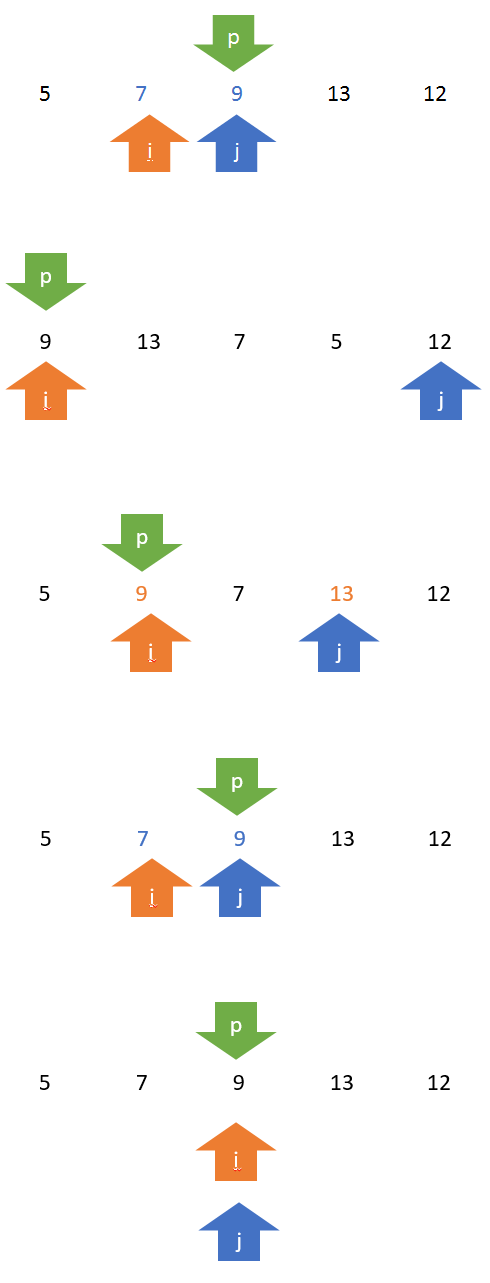BLOG
Enjoy when you can, and endure when you must.AUG 24, 2015/数据结构

Partition 过程p 为主元，即初始时数组中的第一个元素；

i 和 j 的初始状态分别指向数组的最左边和最右边。

```def partition(l, p, r):
"""
PARTITION 过程，实现对子列表 l[p, r] 的原址重排

:param l: 排序列表
:param p: 起始位置
:param r: 终止位置
:return: 主元（pivot）
"""

# 将子列表的第一个元素作为主元，
# 则排序后的列表中主元左侧的元素小于或等于主元，右侧的元素大于或等于主元
pivot = l[p]

left = p
right = r

while left < right:
# 整个查找左右交替进行
# 当从左至右查找，查询比主元小的元素
# 当从右至左查找，则查询比主元大的元素

while left < right and l[right] >= pivot:
right -= 1

l[left], l[right] = l[right], l[left]

while left < right and l[left] <= pivot:
left += 1

l[left], l[right] = l[right], l[left]

# 最后将主元所在的位置返回
return left```

```def quick_sort(l, p=0, r=None):
"""
快速排序

:param l: 排序列表
:param p: 排序起始位置
:param r: 排序终止位置
:return: 无返回
"""

r = len(l) - 1 if r is None else r
if p < r:
# 调用 PARTITION 函数对列表进行划分，并得到划分的支点，即主元所在的位置
q = partition(l, p, r)
# 以主元为中心，对左、后子数组再次排序
quick_sort(l, p, q - 1)
quick_sort(l, q + 1, r)```

《算法导论》第七章

RECOMMENDS